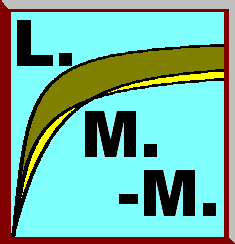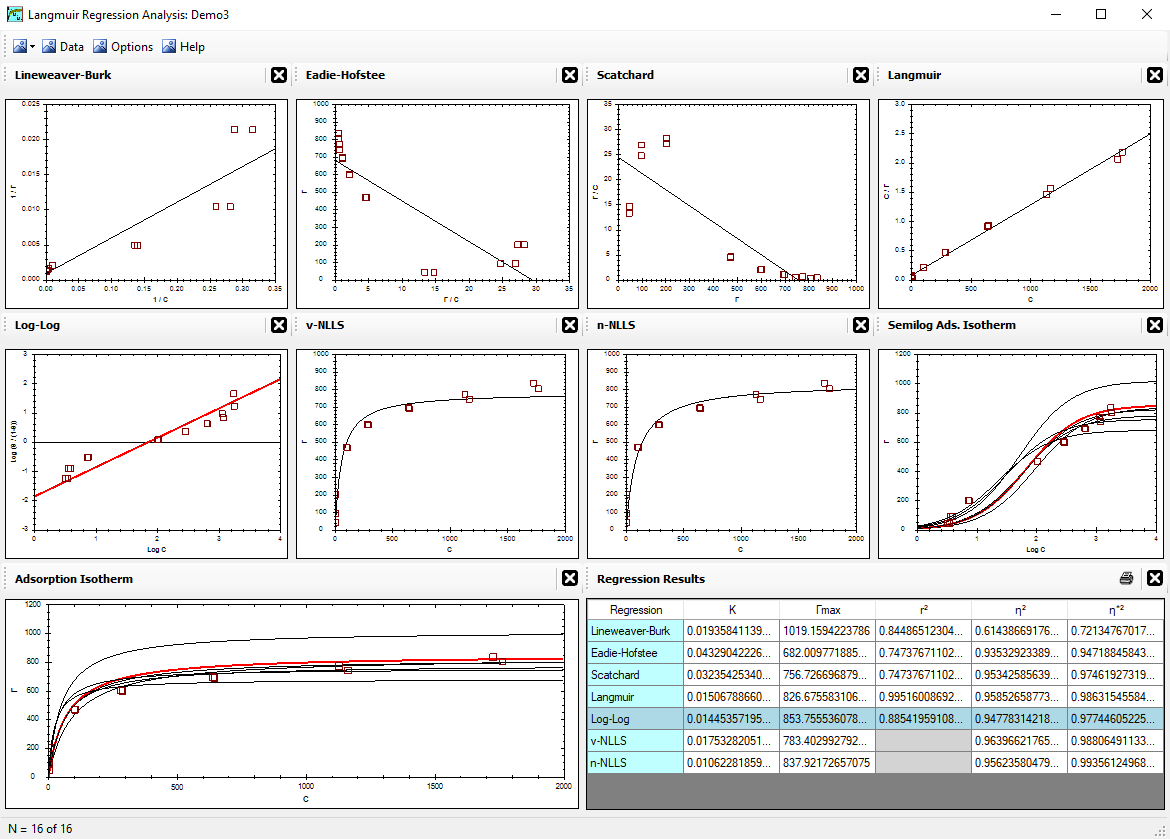Alfisol, Item 003: LMMpro, version 2.0 The Langmuir Optimization Program plus The Michaelis-Menten Optimization ProgramWhat does LMMpro do? List of Published Articles Using LMMpro History of Linear Regression Methods Linear Regressions Deriving Linear Regression Methods

## Deriving Linear Regression Methods

Given a line y = mx + b,
how do we determine what the best line's slope (m) and intercept (b) should be for a given set of data (x,y)?

We could randomly guess the m and b values of the line, tabulate the error of each, and then identify which guess results in the least error. This can be done with a computer, but due to the lack of computers in the late 1700's, an alternate method was proposed that is much better. The method is called a least squares linear regression method because we solve the problem backwards (hence the term "regression"); that is, we first minimize the error and we then find out which line is it that gives us that low error value. The method does not really care what the minimum error value actually is. Instead, we note that the derivative of the error function reaches a minimum and its slope is equal to zero when the error value is also at a minimum.

1. Let ε2 = error2 = Σ (yi - yp)2,
where yp = predicted value when x = xi, and yi = experimental value when x = xi. Note that we work with the square of the error because it eliminates the problem of working with the absolute value of the error. Although the error can be either positive or negative, its square is always positive. It is perfectly reasonable to work with other exponents (say, 4 or 6), but using the power of 2 is easier to solve. This also explains the origin of the name of the method: it's a least squares linear regression.

2. Let yp = m xi + b.

3. Combine (1) and (2), and expand:
ε2 = Σ (yi - m xi - b)2
ε2 = Σ (m2xi2 + 2mbxi - 2mxiyi + b2 - 2byi + yi2)

4. Step 3 above yields a plot of ε2 as a function of the line's slope m and the line's intercept b. As we approach the best value for m and b, ε2 approaches its minimum value. If we overshot the m and b values, the ε2 value begins to increase again. If we stop right at the bottom of the plot, right where the best m and b values exist, then the ε2 is at its minimum and the tangent of this error function right at that point is zero. The tangent at any point in the curve is the first-derivative of the curve. We seek the point where the first-derivative equals zero, and where the error as a function of m and b is at a minimum:
 dε2 dm = 0
, and
 dε2 db = 0

5. Solve Equation  for the minimum as a function of m:
 dε2 dm = 0 = 2mΣxi2 + 2bΣxi - 2Σ(xiyi)

6. Solve Equation  for the minimum as a function of b:
 dε2 db = 0 = 2mΣxi + 2Σb - 2Σyi

7. To simplify the notation (for number of points = n):
Let Sx = Σ xi
Let Sy = Σ yi
Let Sxy = Σ (xiyi)
Let Sxx = Σ (xi2)
Let nb = Σ b

8. From step 5, solve for m:
 m = Sxy - b Sx Sxx

Or, stated differently, Sxy = mSxx + bSx.

We will use this optimization of slope in step 10 below, where it is used in combination with the intercept optimization. Do not use this equation to solve for m when the intercept's value b is fixed. Similarly, do not rearrange this equation to solve for b when the slope m is fixed. For these situations, use step 9 instead. Although by definition the sum of the square of the errors is minimized in step 5, optimizing the slope in step 5 does not necessarily result in a regression line whose sum of the errors is also zero because here a zero value for the sum of the errors depends on b.

9. From step 6, solve for b:

b = (Sy - m Sx) / n .

Or, stated differently, Sy = mSx + nb.

If the slope's value m is fixed, then all parameters are known, and solve for b. If the intercept's value b is fixed, rearrange this equation, and solve for m. Although by definition the sum of the square of the errors is minimized in step 6, it is important to note that this time it also results in a regression line whose sum of the errors is zero for any value of b or m chosen. That is, the regression line resulting from step 6 will be arithmetically balanced as long as one of the two parameters (b or m) can be adjusted.

10. If neither the slope nor the intercept are known, then combine step 9 and 8, and solve for m:

0 = m Sxx + Sx (Sy - m Sx)/n - Sxy

0 = m Sxx + SxSy/n - m SxSx/n - Sxy

0 = mn Sxx + SxSy - m SxSx - n Sxy

m (n Sxx - SxSx) = n Sxy - SxSy

 m = n Sxy - Sx Sy n Sxx - Sx Sx

Now plug this optimized value of m into step 9 to get the optimized value of b. This regression line will have a minimum value for the sum of the square of the errors, and a zero value for the sum of the errors.

This regression analysis is easy enough to do by hand. It is even easier today with computers that do it for us.